ROOT   Reference Guide
Searching...
No Matches
TLatex Class Reference

To draw Mathematical Formula.

TLatex's purpose is to write mathematical equations. The syntax is very similar to the Latex's one. It provides several functionalities:

When the font precision (see TAttText) is low (0 or 1), TLatex is painted as a normal TText, the control characters are not interpreted.

## Subscripts and Superscripts

Subscripts and superscripts are made with the _ and ^ commands. These commands can be combined to make complicated subscript and superscript expressions. You may adjust the display of subscripts and superscripts by using the two functions SetIndiceSize(Double_t), which set relative size of subscripts and superscripts, and SetLimitIndiceSize(Int_t), which set limits for text resizing of subscripts and superscripts.

Examples: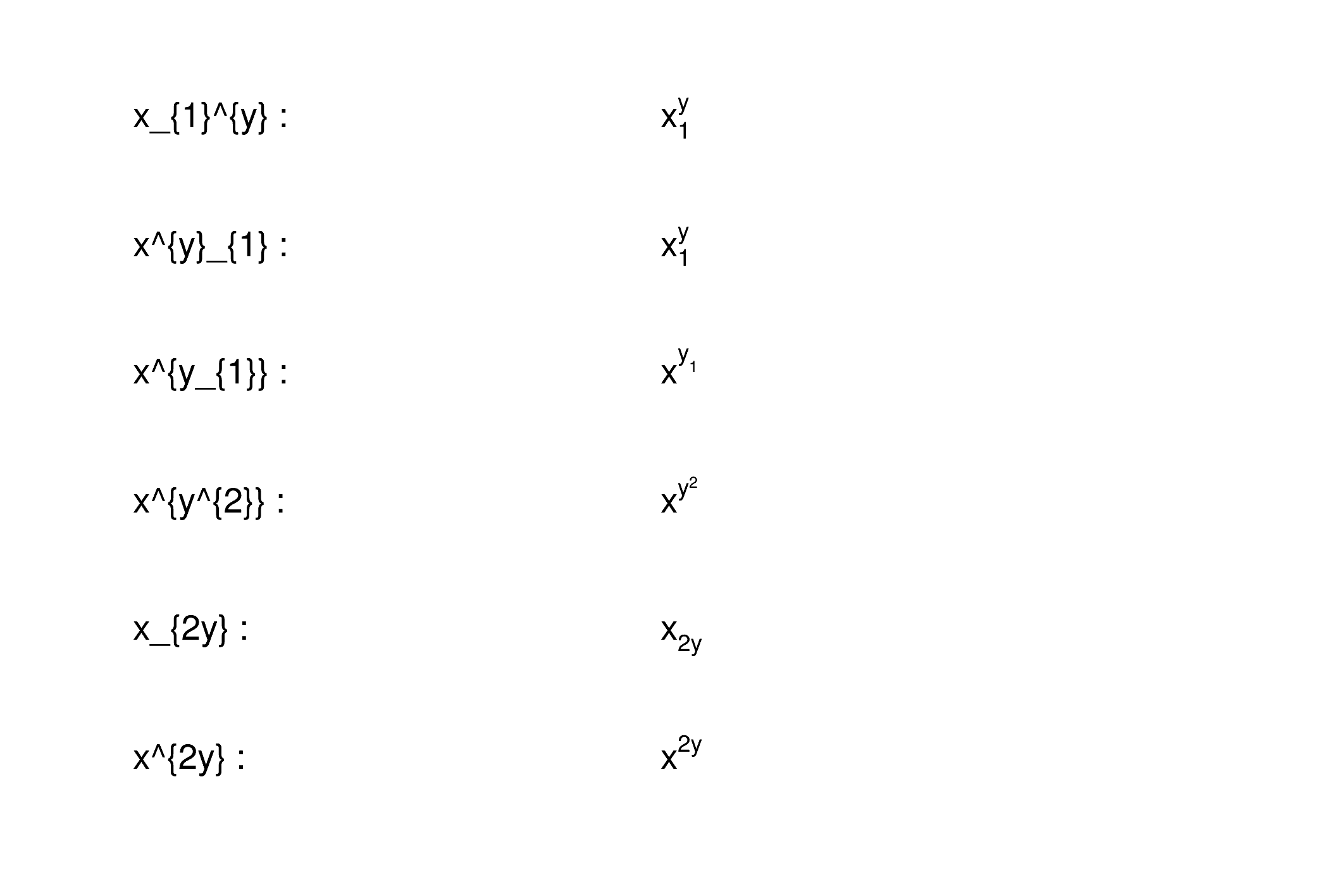The best way to put the subscripts and superscripts before the character and not after, is to use an empty character:The subscripts and superscripts operators apply not only on one character but on all the "normal text" preceding them. In the following example the second E is lower than the first one because the operator _ is applied on /f which has a descending part, and not only on f which as no descending part.To make sure the second operator _ applies only on f a dummy operator ^{} should be introduced to separate the f from the /.## Fractions

Fractions denoted by the / symbol are made in the obvious way. The #frac command is used for large fractions in displayed formula; it has two arguments: the numerator and the denominator.

Examples:## Splitting Lines

Text can be split in two lines via the command #splitline.

Examples:## Roots

The #sqrt command produces the square root of its argument; it has an optional first argument for other roots.

Examples:## Mathematical Symbols

TLatex can display dozens of special mathematical symbols. A few of them, such as + and > , are produced by typing the corresponding keyboard character. Others are obtained with the commands in the following table: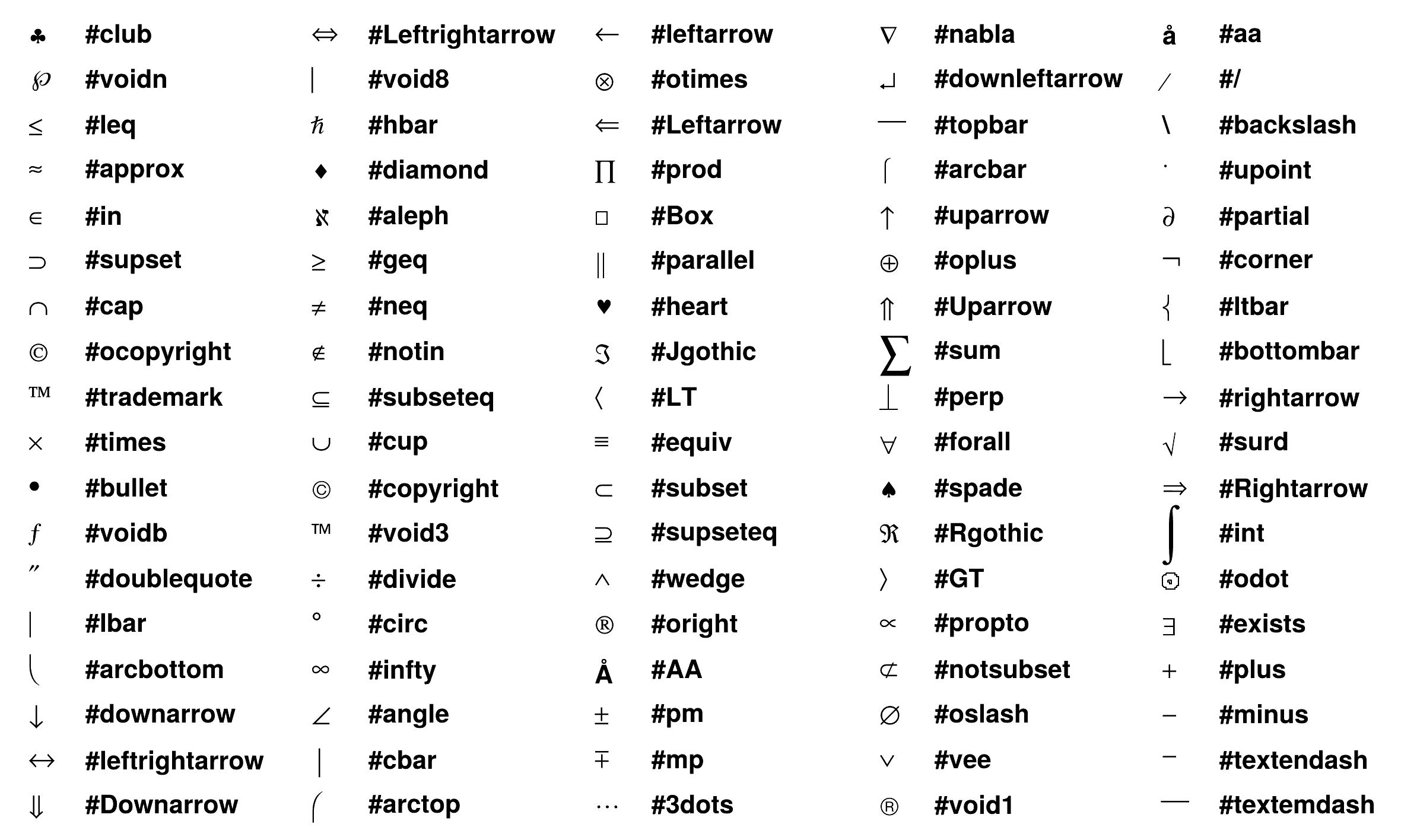## Delimiters

TLatex provides 4 kinds of proportional delimiters:

#[]{....} or "a la" Latex #left[.....#right] : big square brackets
#{}{....} or              #left{.....#right} : big curly brackets
#||{....} or              #left|.....#right| : big absolute value symbols
#(){....} or              #left(.....#right) : big parentheses


## Greek Letters

The command to produce a lowercase Greek letter is obtained by adding a # to the name of the letter. For an uppercase Greek letter, just capitalize the first letter of the command name. Some letters have two representations. The name of the second one (the "variation") starts with "var". The following table gives the complete list: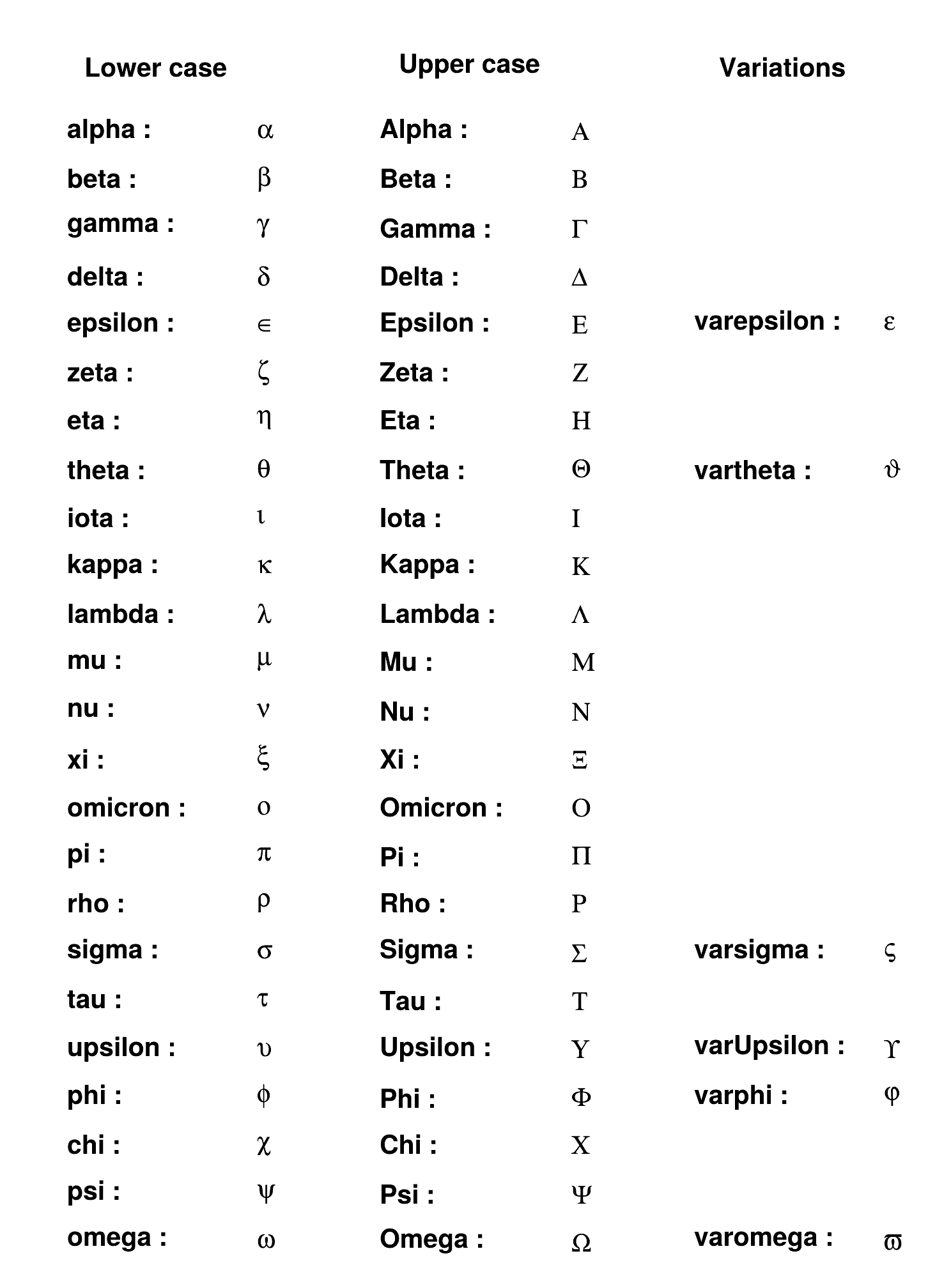## Accents

Several kind of accents are available: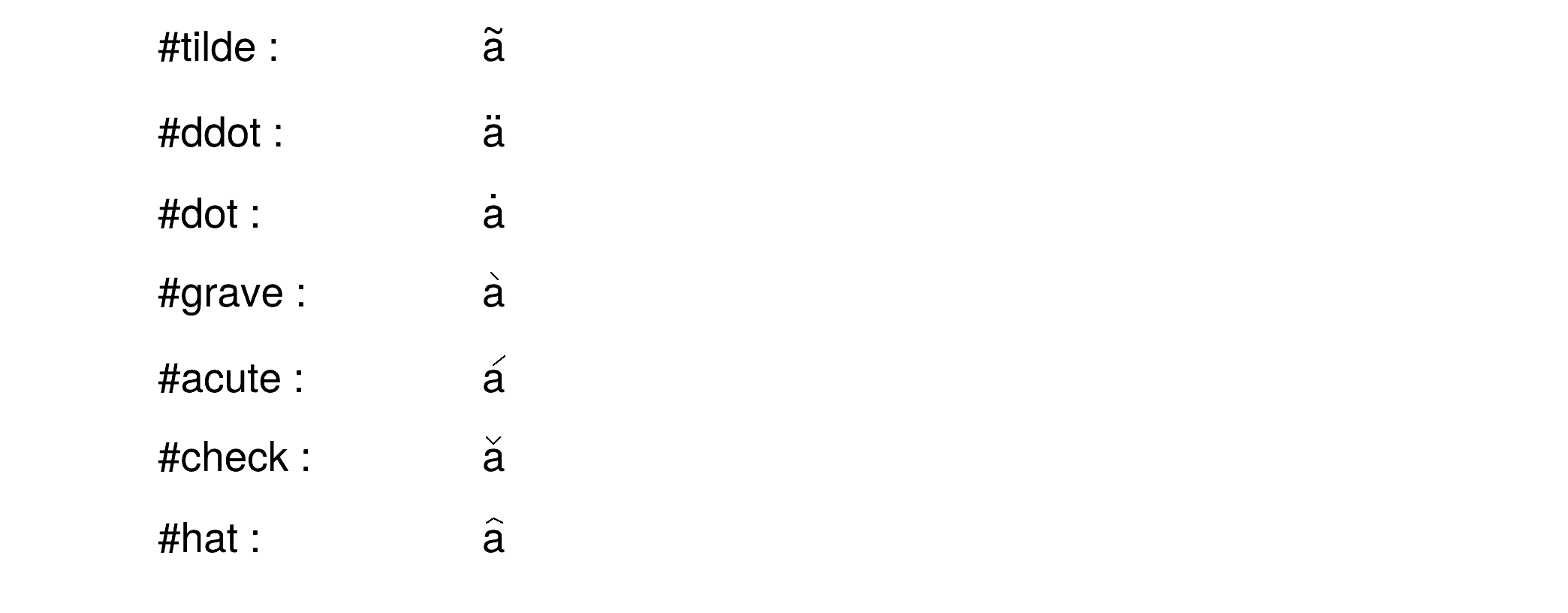The special sign: #slash draws a slash on top of the text between brackets:Bar and vectors sign are done the following way:## Changing Style

One can change the font, the text color, or the text size at any time using : #font[font-number]{...}, #color[color-number]{...} and #scale[scale-factor]{...}

Examples: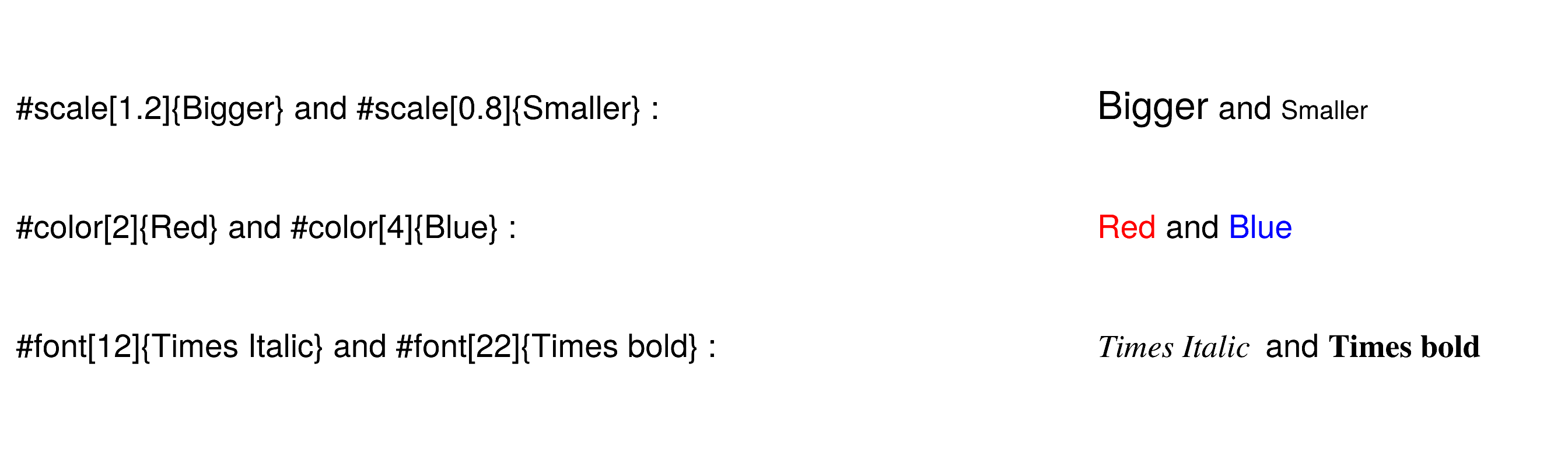## Alignment Rules

The TText alignment rules apply to the TLatex objects with one exception concerning the vertical alignment:

• if the vertical alignment = 1 , subscripts are not taken into account
• if the vertical alignment = 0 , the text is aligned to the box surrounding the full text with sub and superscripts

This is illustrated by the following example:

{
TCanvas Tlva("Tlva","Tlva",500,500);
Tlva.SetGrid();
Tlva.DrawFrame(0,0,1,1);
const char *longstring = "K_{S}... K^{*0}... #frac{2s}{#pi#alpha^{2}} #frac{d#sigma}{dcos#theta} (e^{+}e^{-} #rightarrow f#bar{f} ) = #left| #frac{1}{1 - #Delta#alpha} #right|^{2} (1+cos^{2}#theta)";
TLatex latex;
latex.SetTextSize(0.025);
latex.SetTextAlign(13); //align at top
latex.DrawLatex(.2,.9,"K_{S}");
latex.DrawLatex(.3,.9,"K^{*0}");
latex.DrawLatex(.2,.8,longstring);
latex.SetTextAlign(12); //centered
latex.DrawLatex(.2,.6,"K_{S}");
latex.DrawLatex(.3,.6,"K^{*0}");
latex.DrawLatex(.2,.5,longstring);
latex.SetTextAlign(11); //default bottom alignment
latex.DrawLatex(.2,.4,"K_{S}");
latex.DrawLatex(.3,.4,"K^{*0}");
latex.DrawLatex(.2,.3,longstring);
latex.SetTextAlign(10); //special bottom alignment
latex.DrawLatex(.2,.2,"K_{S}");
latex.DrawLatex(.3,.2,"K^{*0}");
latex.DrawLatex(.2,.1,longstring);
latex.SetTextAlign(12);
latex.SetTextFont(72);
latex.DrawLatex(.1,.80,"13");
latex.DrawLatex(.1,.55,"12");
latex.DrawLatex(.1,.35,"11");
latex.DrawLatex(.1,.18,"10");
return Tlva;
}
virtual void SetTextAlign(Short_t align=11)
Set the text alignment.
Definition TAttText.h:42
virtual void SetTextFont(Font_t tfont=62)
Set the text font.
Definition TAttText.h:46
virtual void SetTextSize(Float_t tsize=1)
Set the text size.
Definition TAttText.h:47
The Canvas class.
Definition TCanvas.h:23
To draw Mathematical Formula.
Definition TLatex.h:18
TLatex * DrawLatex(Double_t x, Double_t y, const char *text)
Make a copy of this object with the new parameters And copy object attributes.
Definition TLatex.cxx:1928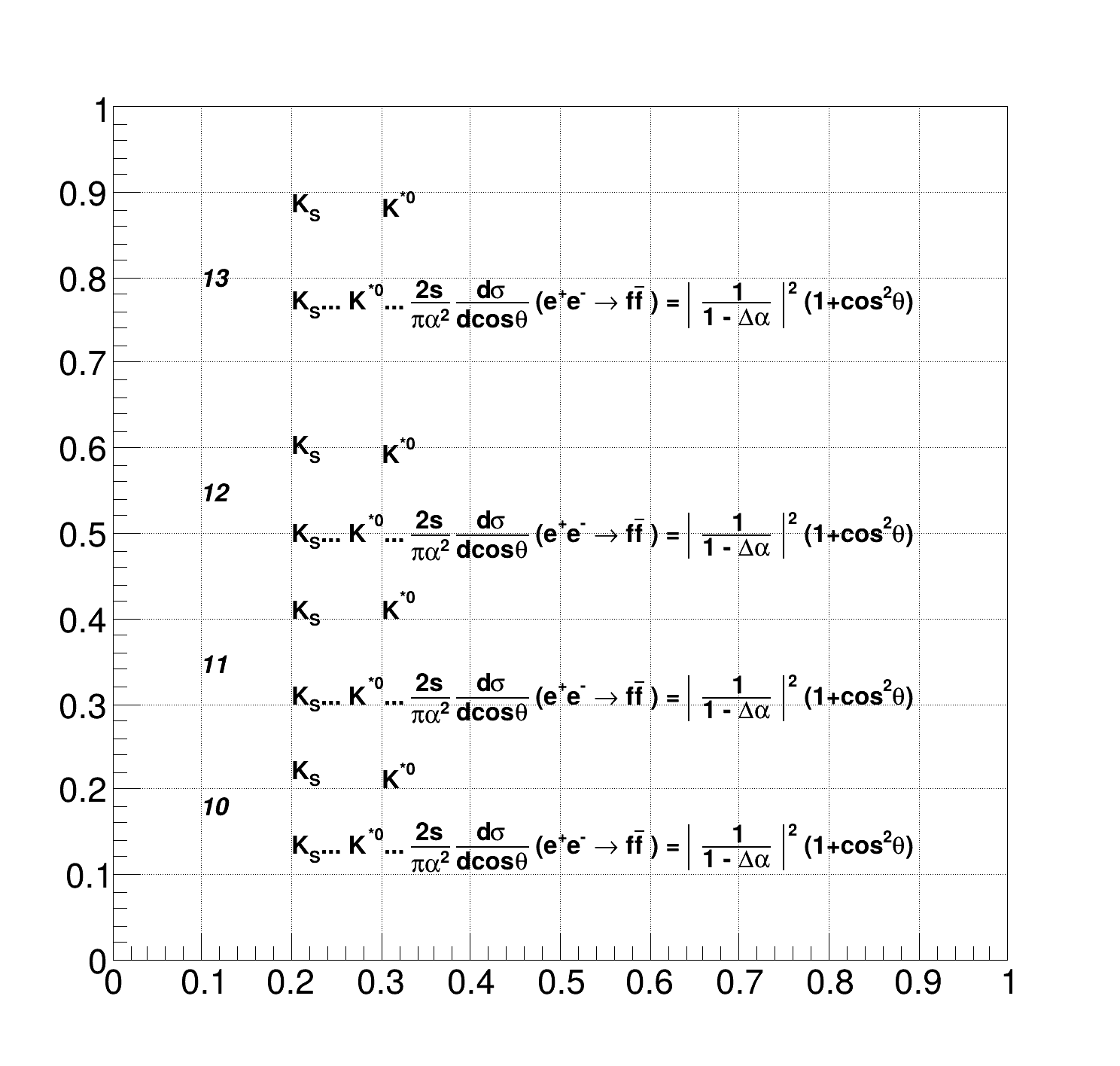The two commands #kern and #lower enable a better control over character placement. The command #kern[(Float_t)dx]{text} moves the output string horizontally by the fraction dx of its length. Similarly, #lower[(Float_t)dy]{text} shifts the text up or down by the fraction dy of its height.

Examples: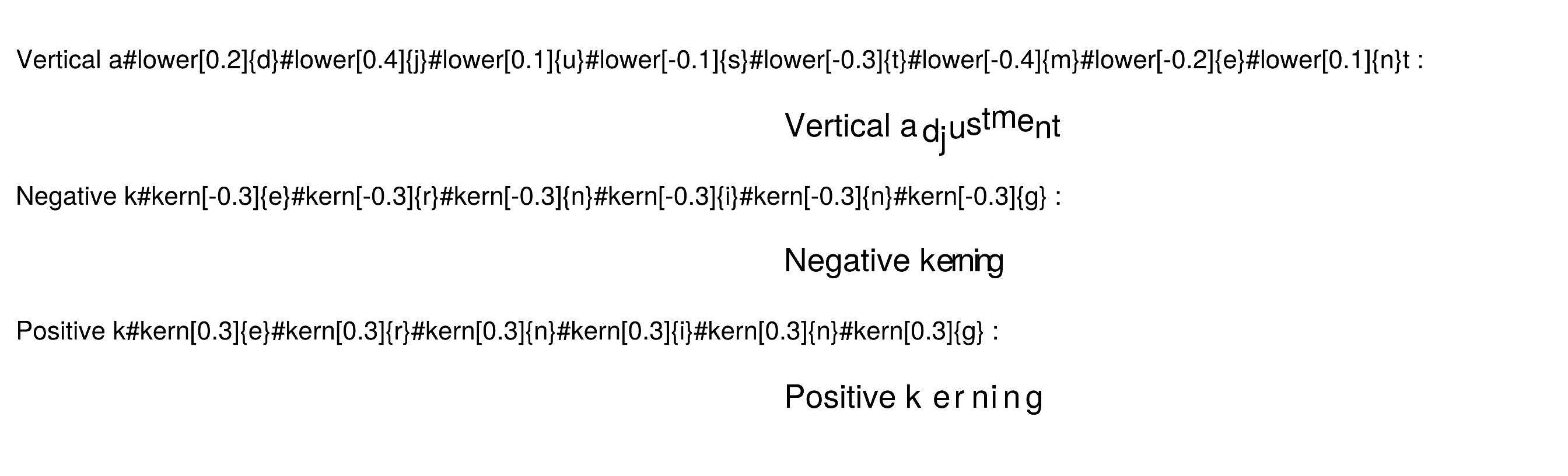## Italic and Boldface

Text can be turned italic or boldface using the commands #it and #bf.

Examples: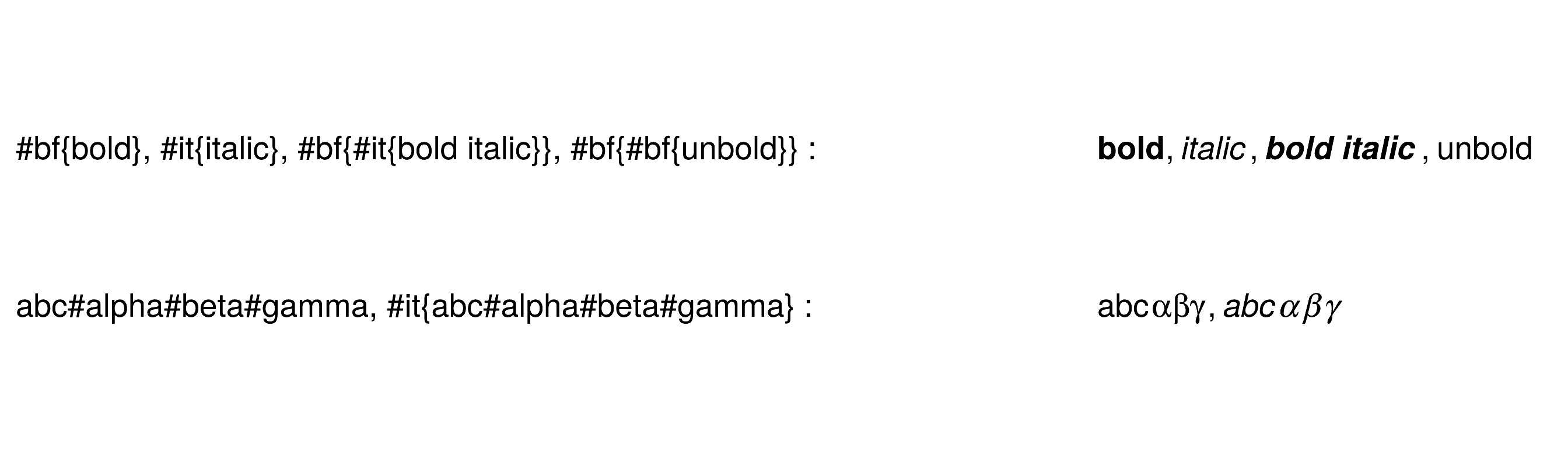## Examples

{
TCanvas ex1("ex1","Latex",500,600);
TLatex Tl;
Tl.SetTextAlign(12);
Tl.SetTextSize(0.04);
Tl.DrawLatex(0.1,0.8,"1) C(x) = d #sqrt{#frac{2}{#lambdaD}} #int^{x}_{0}cos(#frac{#pi}{2}t^{2})dt");
Tl.DrawLatex(0.1,0.6,"2) C(x) = d #sqrt{#frac{2}{#lambdaD}} #int^{x}cos(#frac{#pi}{2}t^{2})dt");
Tl.DrawLatex(0.1,0.4,"3) R = |A|^{2} = #frac{1}{2}(#[]{#frac{1}{2}+C(V)}^{2}+#[]{#frac{1}{2}+S(V)}^{2})");
Tl.DrawLatex(0.1,0.2,"4) F(t) = #sum_{i=-#infty}^{#infty}A(i)cos#[]{#frac{i}{t+i}}");
return ex1;
}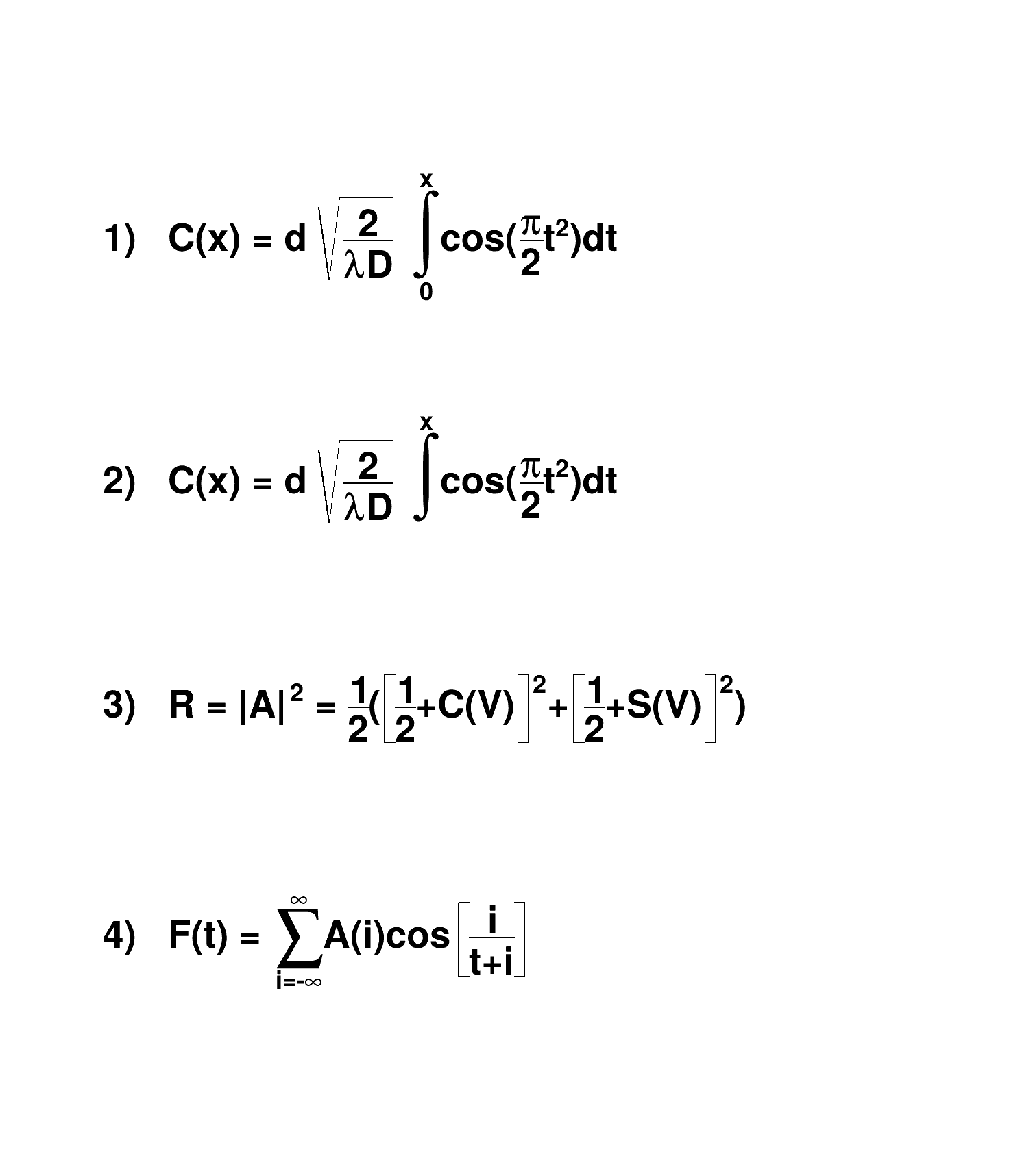{
TCanvas ex2("ex2","Latex",500,300);
TLatex Tl;
Tl.SetTextAlign(23);
Tl.SetTextSize(0.08);
Tl.DrawLatex(0.5,0.95,"e^{+}e^{-}#rightarrowZ^{0}#rightarrowI#bar{I}, q#bar{q}");
Tl.DrawLatex(0.5,0.75,"|#vec{a}#bullet#vec{b}|=#Sigmaa^{i}_{jk}+b^{bj}_{i}");
Tl.DrawLatex(0.5,0.5,"i(#partial_{#mu}#bar{#psi}#gamma^{#mu}+m#bar{#psi}=0#Leftrightarrow(#Box+m^{2})#psi=0");
Tl.DrawLatex(0.5,0.3,"L_{em}=eJ^{#mu}_{em}A_{#mu} , J^{#mu}_{em}=#bar{I}#gamma_{#mu}I , M^{j}_{i}=#SigmaA_{#alpha}#tau^{#alphaj}_{i}");
return ex2;
}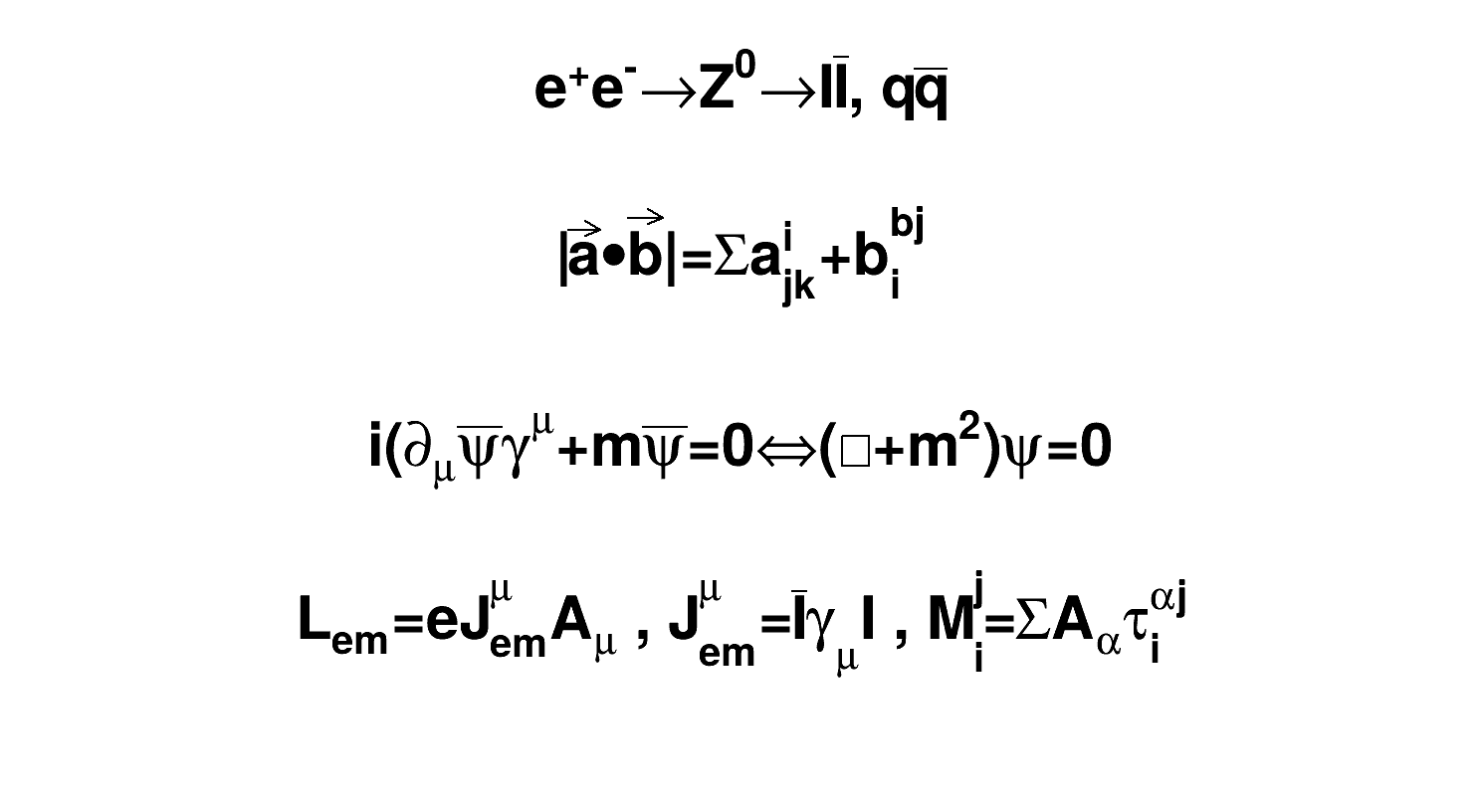{
TCanvas ex3("ex3","Latex",500,300);
TPaveText pt(.1,.1,.9,.9);
pt.AddText("#frac{2s}{#pi#alpha^{2}} #frac{d#sigma}{dcos#theta} (e^{+}e^{-} #rightarrow f#bar{f} ) = ");
pt.AddText("#left| #frac{1}{1 - #Delta#alpha} #right|^{2} (1+cos^{2}#theta");
pt.AddText("+ 4 Re #left{ #frac{2}{1 - #Delta#alpha} #chi(s) #[]{#hat{g}_{#nu}^{e}#hat{g}_{#nu}^{f} (1 + cos^{2}#theta) + 2 #hat{g}_{a}^{e}#hat{g}_{a}^{f} cos#theta) } #right}");
pt.SetLabel("Born equation");
pt.Draw();
return ex3;
}
A Pave (see TPave) with text, lines or/and boxes inside.
Definition TPaveText.h:21
virtual TText * AddText(Double_t x1, Double_t y1, const char *label)
Add a new Text line to this pavetext at given coordinates.
virtual void SetLabel(const char *label)
Definition TPaveText.h:61
void Draw(Option_t *option="") override
Draw this pavetext with its current attributes.
TPaveText * pt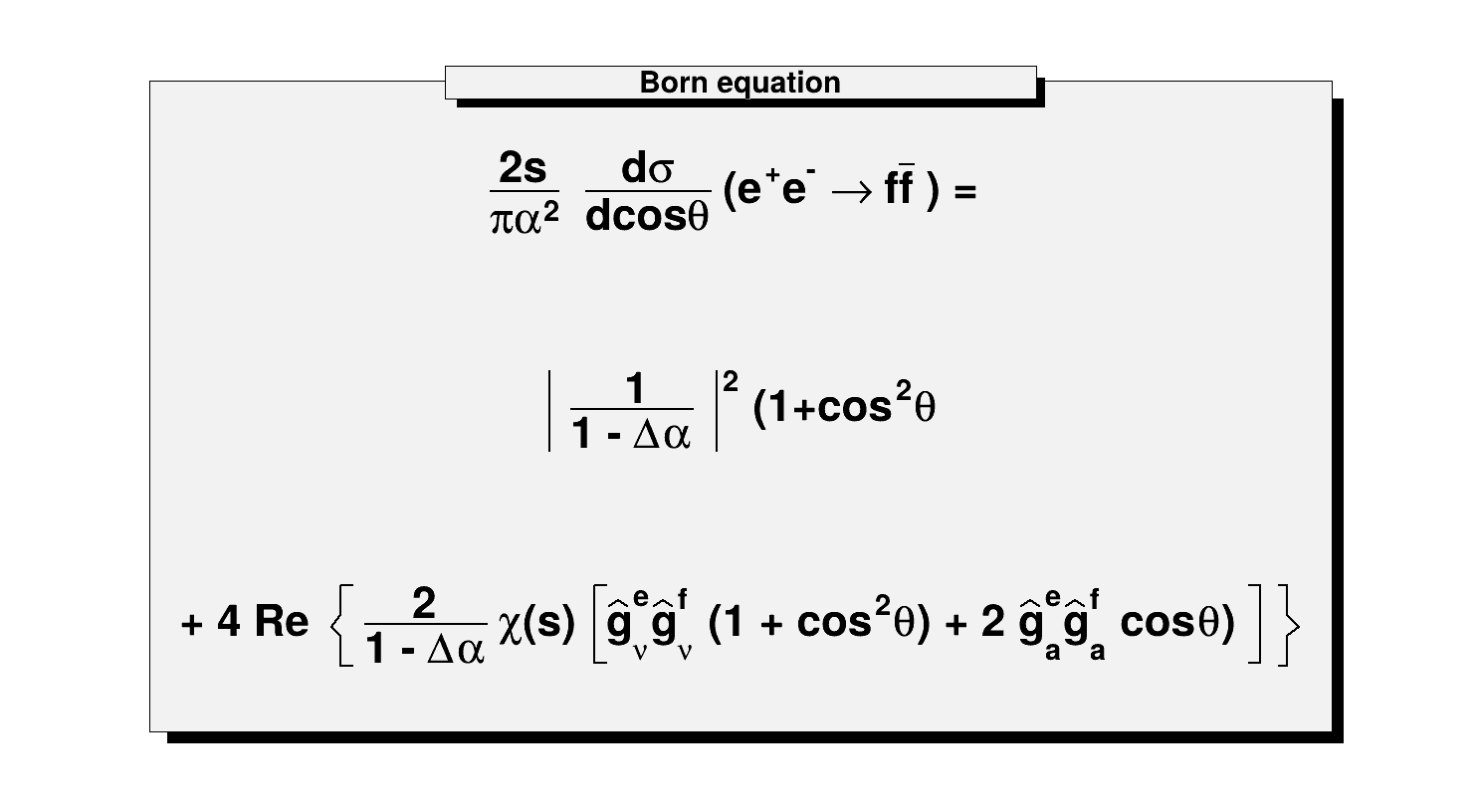## Interface to TMathText

The class TMathText is a TeX math formulae interpreter. It uses plain TeX syntax and uses "\\" as control instead of "#". If a piece of text containing "\" is given to TLatex then TMathText is automatically invoked. Therefore, as histograms' titles, axis titles, labels etc ... are drawn using TLatex, the TMathText syntax can be used for them also.

Definition at line 18 of file TLatex.h.

## Classes

struct  TextSpec_t
TLatex helper struct holding the attributes of a piece of text. More...

class  TLatexFormSize
TLatex helper class used to compute the size of a portion of a formula. More...

## Public Types

enum  { kTextNDC = (1ULL << ( 14 )) }Public Types inherited from TText
enum  { kTextNDC = (1ULL << ( 14 )) }Public Types inherited from TObject
enum  {
kIsOnHeap = 0x01000000 , kNotDeleted = 0x02000000 , kZombie = 0x04000000 , kInconsistent = 0x08000000 ,
}

enum  { kSingleKey = (1ULL << ( 0 )) , kOverwrite = (1ULL << ( 1 )) , kWriteDelete = (1ULL << ( 2 )) }

enum  EDeprecatedStatusBits { kObjInCanvas = (1ULL << ( 3 )) }

enum  EStatusBits {
kCanDelete = (1ULL << ( 0 )) , kMustCleanup = (1ULL << ( 3 )) , kIsReferenced = (1ULL << ( 4 )) , kHasUUID = (1ULL << ( 5 )) ,
kCannotPick = (1ULL << ( 6 )) , kNoContextMenu = (1ULL << ( 8 )) , kInvalidObject = (1ULL << ( 13 ))
}

## Public Member Functions

TLatex ()
Default constructor.

TLatex (const TLatex &text)
Copy constructor.

TLatex (Double_t x, Double_t y, const char *text)
Normal constructor.

~TLatex () override
Destructor.

void Copy (TObject &text) const override
Copy this TLatex object to another TLatex.

TLatexDrawLatex (Double_t x, Double_t y, const char *text)
Make a copy of this object with the new parameters And copy object attributes.

TLatexDrawLatexNDC (Double_t x, Double_t y, const char *text)
Draw this TLatex with new coordinates in NDC.

void GetBoundingBox (UInt_t &w, UInt_t &h, Bool_t angle=kFALSE) override
Return text size in pixels.

Double_t GetHeight () const
Return height of current pad in pixels.

Double_t GetXsize ()
Return size of the formula along X in pad coordinates when the text precision is smaller than 3.

Double_t GetYsize ()
Return size of the formula along Y in pad coordinates when the text precision is smaller than 3.

TClassIsA () const override

void Paint (Option_t *option="") override
Paint.

virtual void PaintLatex (Double_t x, Double_t y, Double_t angle, Double_t size, const char *text)
Main drawing function.

void SavePrimitive (std::ostream &out, Option_t *option="") override
Save primitive as a C++ statement(s) on output stream out.

virtual void SetIndiceSize (Double_t factorSize)
Set relative size of subscripts and superscripts.

virtual void SetLimitIndiceSize (Int_t limitFactorSize)
Set limit for text resizing of subscripts and superscripts.

void Streamer (TBuffer &) override
Stream an object of class TObject.

void StreamerNVirtual (TBuffer &ClassDef_StreamerNVirtual_b)Public Member Functions inherited from TText
TText ()

TText (const TText &text)
Copy constructor.

TText (Double_t x, Double_t y, const char *text)
Text normal constructor.

TText (Double_t x, Double_t y, const wchar_t *text)
Text normal constructor.

~TText () override
Text default destructor.

void Copy (TObject &text) const override
Copy this text to text.

Int_t DistancetoPrimitive (Int_t px, Int_t py) override
Compute distance from point px,py to a string.

virtual TTextDrawText (Double_t x, Double_t y, const char *text)
Draw this text with new coordinates.

virtual TTextDrawText (Double_t x, Double_t y, const wchar_t *text)
Draw this text with new coordinates.

virtual TTextDrawTextNDC (Double_t x, Double_t y, const char *text)
Draw this text with new coordinates in NDC.

virtual TTextDrawTextNDC (Double_t x, Double_t y, const wchar_t *text)
Draw this text with new coordinates in NDC.

void ExecuteEvent (Int_t event, Int_t px, Int_t py) override
Execute action corresponding to one event.

Rectangle_t GetBBox () override
Return the "bounding Box" of the Box.

TPoint GetBBoxCenter () override
Return the point given by Alignment as 'center'.

virtual void GetControlBox (Int_t x, Int_t y, Double_t theta, Int_t cBoxX, Int_t cBoxY)
Return the text control box.

virtual void GetTextAdvance (UInt_t &a, const char *text, const Bool_t kern=kTRUE) const
Return text advance for string text if kern is true (default) kerning is taken into account.

virtual void GetTextAscentDescent (UInt_t &a, UInt_t &d, const char *text) const
Return text ascent and descent for string text.

virtual void GetTextAscentDescent (UInt_t &a, UInt_t &d, const wchar_t *text) const
Return text ascent and descent for string text.

virtual void GetTextExtent (UInt_t &w, UInt_t &h, const char *text) const
Return text extent for string text.

virtual void GetTextExtent (UInt_t &w, UInt_t &h, const wchar_t *text) const
Return text extent for string text.

const void * GetWcsTitle (void) const
Returns the text as UNICODE.

Double_t GetX () const

Double_t GetY () const

TClassIsA () const override

void ls (Option_t *option="") const override
List this text with its attributes.

TTextoperator= (const TText &src)
Assignment operator.

void Paint (Option_t *option="") override
Paint this text with its current attributes.

virtual void PaintControlBox (Int_t x, Int_t y, Double_t theta)
Paint the text control box.

virtual void PaintText (Double_t x, Double_t y, const char *text)
Draw this text with new coordinates.

virtual void PaintText (Double_t x, Double_t y, const wchar_t *text)
Draw this text with new coordinates.

virtual void PaintTextNDC (Double_t u, Double_t v, const char *text)
Draw this text with new coordinates in NDC.

virtual void PaintTextNDC (Double_t u, Double_t v, const wchar_t *text)
Draw this text with new coordinates in NDC.

void Print (Option_t *option="") const override
Dump this text with its attributes.

void SavePrimitive (std::ostream &out, Option_t *option="") override
Save primitive as a C++ statement(s) on output stream out.

void SetBBoxCenter (const TPoint &p) override
Set the point given by Alignment as 'center'.

void SetBBoxCenterX (const Int_t x) override
Set X coordinate of the point given by Alignment as 'center'.

void SetBBoxCenterY (const Int_t y) override
Set Y coordinate of the point given by Alignment as 'center'.

void SetBBoxX1 (const Int_t) override
Set left hand side of BoundingBox to a value (resize in x direction on left)

void SetBBoxX2 (const Int_t) override
Set right hand side of BoundingBox to a value (resize in x direction on right)

void SetBBoxY1 (const Int_t) override
Set top of BoundingBox to a value (resize in y direction on top)

void SetBBoxY2 (const Int_t) override
Set bottom of BoundingBox to a value (resize in y direction on bottom)

virtual void SetMbTitle (const wchar_t *title=L"")
Change (i.e. set) the title of the TNamed.

virtual void SetNDC (Bool_t isNDC=kTRUE)
Set NDC mode on if isNDC = kTRUE, off otherwise.

virtual void SetText (Double_t x, Double_t y, const char *text)

virtual void SetText (Double_t x, Double_t y, const wchar_t *text)

virtual void SetX (Double_t x)

virtual void SetY (Double_t y)

void Streamer (TBuffer &) override
Stream an object of class TText.

void StreamerNVirtual (TBuffer &ClassDef_StreamerNVirtual_b)Public Member Functions inherited from TNamed
TNamed ()

TNamed (const char *name, const char *title)

TNamed (const TNamed &named)
TNamed copy ctor.

TNamed (const TString &name, const TString &title)

virtual ~TNamed ()
TNamed destructor.

void Clear (Option_t *option="") override
Set name and title to empty strings ("").

TObjectClone (const char *newname="") const override
Make a clone of an object using the Streamer facility.

Int_t Compare (const TObject *obj) const override
Compare two TNamed objects.

virtual void FillBuffer (char *&buffer)
Encode TNamed into output buffer.

const char * GetName () const override
Returns name of object.

const char * GetTitle () const override
Returns title of object.

ULong_t Hash () const override
Return hash value for this object.

Bool_t IsSortable () const override

TNamedoperator= (const TNamed &rhs)
TNamed assignment operator.

virtual void SetName (const char *name)
Set the name of the TNamed.

virtual void SetNameTitle (const char *name, const char *title)
Set all the TNamed parameters (name and title).

virtual void SetTitle (const char *title="")
Set the title of the TNamed.

virtual Int_t Sizeof () const
Return size of the TNamed part of the TObject.

void StreamerNVirtual (TBuffer &ClassDef_StreamerNVirtual_b)Public Member Functions inherited from TObject
TObject ()
TObject constructor.

TObject (const TObject &object)
TObject copy ctor.

virtual ~TObject ()
TObject destructor.

void AbstractMethod (const char *method) const
Use this method to implement an "abstract" method that you don't want to leave purely abstract.

Append graphics object to current pad.

virtual void Browse (TBrowser *b)
Browse object. May be overridden for another default action.

ULong_t CheckedHash ()
Check and record whether this class has a consistent Hash/RecursiveRemove setup (*) and then return the regular Hash value for this object.

virtual const char * ClassName () const
Returns name of class to which the object belongs.

virtual void Delete (Option_t *option="")
Delete this object.

virtual void Draw (Option_t *option="")
Default Draw method for all objects.

virtual void DrawClass () const
Draw class inheritance tree of the class to which this object belongs.

virtual TObjectDrawClone (Option_t *option="") const
Draw a clone of this object in the current selected pad with: gROOT->SetSelectedPad(c1).

virtual void Dump () const
Dump contents of object on stdout.

virtual void Error (const char *method, const char *msgfmt,...) const
Issue error message.

virtual void Execute (const char *method, const char *params, Int_t *error=nullptr)
Execute method on this object with the given parameter string, e.g.

virtual void Execute (TMethod *method, TObjArray *params, Int_t *error=nullptr)
Execute method on this object with parameters stored in the TObjArray.

virtual void Fatal (const char *method, const char *msgfmt,...) const
Issue fatal error message.

virtual TObjectFindObject (const char *name) const
Must be redefined in derived classes.

virtual TObjectFindObject (const TObject *obj) const
Must be redefined in derived classes.

virtual Option_tGetDrawOption () const
Get option used by the graphics system to draw this object.

virtual const char * GetIconName () const
Returns mime type name of object.

virtual char * GetObjectInfo (Int_t px, Int_t py) const
Returns string containing info about the object at position (px,py).

virtual Option_tGetOption () const

virtual UInt_t GetUniqueID () const
Return the unique object id.

virtual Bool_t HandleTimer (TTimer *timer)
Execute action in response of a timer timing out.

Bool_t HasInconsistentHash () const
Return true is the type of this object is known to have an inconsistent setup for Hash and RecursiveRemove (i.e.

virtual void Info (const char *method, const char *msgfmt,...) const
Issue info message.

virtual Bool_t InheritsFrom (const char *classname) const
Returns kTRUE if object inherits from class "classname".

virtual Bool_t InheritsFrom (const TClass *cl) const
Returns kTRUE if object inherits from TClass cl.

virtual void Inspect () const
Dump contents of this object in a graphics canvas.

void InvertBit (UInt_t f)

Bool_t IsDestructed () const
IsDestructed.

virtual Bool_t IsEqual (const TObject *obj) const
Default equal comparison (objects are equal if they have the same address in memory).

virtual Bool_t IsFolder () const
Returns kTRUE in case object contains browsable objects (like containers or lists of other objects).

R__ALWAYS_INLINE Bool_t IsOnHeap () const

R__ALWAYS_INLINE Bool_t IsZombie () const

void MayNotUse (const char *method) const
Use this method to signal that a method (defined in a base class) may not be called in a derived class (in principle against good design since a child class should not provide less functionality than its parent, however, sometimes it is necessary).

virtual Bool_t Notify ()
This method must be overridden to handle object notification (the base implementation is no-op).

void Obsolete (const char *method, const char *asOfVers, const char *removedFromVers) const
Use this method to declare a method obsolete.

void operator delete (void *ptr)
Operator delete.

void operator delete[] (void *ptr)
Operator delete [].

void * operator new (size_t sz)

void * operator new (size_t sz, void *vp)

void * operator new[] (size_t sz)

void * operator new[] (size_t sz, void *vp)

TObjectoperator= (const TObject &rhs)
TObject assignment operator.

virtual void Pop ()
Pop on object drawn in a pad to the top of the display list.

virtual Int_t Read (const char *name)
Read contents of object with specified name from the current directory.

virtual void RecursiveRemove (TObject *obj)
Recursively remove this object from a list.

void ResetBit (UInt_t f)

virtual void SaveAs (const char *filename="", Option_t *option="") const
Save this object in the file specified by filename.

void SetBit (UInt_t f)

void SetBit (UInt_t f, Bool_t set)
Set or unset the user status bits as specified in f.

virtual void SetDrawOption (Option_t *option="")
Set drawing option for object.

virtual void SetUniqueID (UInt_t uid)
Set the unique object id.

void StreamerNVirtual (TBuffer &ClassDef_StreamerNVirtual_b)

virtual void SysError (const char *method, const char *msgfmt,...) const
Issue system error message.

R__ALWAYS_INLINE Bool_t TestBit (UInt_t f) const

Int_t TestBits (UInt_t f) const

virtual void UseCurrentStyle ()
Set current style settings in this object This function is called when either TCanvas::UseCurrentStyle or TROOT::ForceStyle have been invoked.

virtual void Warning (const char *method, const char *msgfmt,...) const
Issue warning message.

virtual Int_t Write (const char *name=nullptr, Int_t option=0, Int_t bufsize=0)
Write this object to the current directory.

virtual Int_t Write (const char *name=nullptr, Int_t option=0, Int_t bufsize=0) const
Write this object to the current directory.Public Member Functions inherited from TAttText
TAttText ()
AttText default constructor.

TAttText (Int_t align, Float_t angle, Color_t color, Style_t font, Float_t tsize)
AttText normal constructor.

virtual ~TAttText ()
AttText destructor.

void Copy (TAttText &atttext) const
Copy this text attributes to a new TAttText.

virtual Short_t GetTextAlign () const
Return the text alignment.

virtual Float_t GetTextAngle () const
Return the text angle.

virtual Color_t GetTextColor () const
Return the text color.

virtual Font_t GetTextFont () const
Return the text font.

virtual Float_t GetTextSize () const
Return the text size.

virtual Float_t GetTextSizePercent (Float_t size)
Return the text in percent of the pad size.

virtual void Modify ()
Change current text attributes if necessary.

virtual void ResetAttText (Option_t *toption="")
Reset this text attributes to default values.

virtual void SaveTextAttributes (std::ostream &out, const char *name, Int_t alidef=12, Float_t angdef=0, Int_t coldef=1, Int_t fondef=61, Float_t sizdef=1)
Save text attributes as C++ statement(s) on output stream out.

virtual void SetTextAlign (Short_t align=11)
Set the text alignment.

virtual void SetTextAngle (Float_t tangle=0)
Set the text angle.

virtual void SetTextAttributes ()
Invoke the DialogCanvas Text attributes.

virtual void SetTextColor (Color_t tcolor=1)
Set the text color.

virtual void SetTextColorAlpha (Color_t tcolor, Float_t talpha)
Set a transparent marker color.

virtual void SetTextFont (Font_t tfont=62)
Set the text font.

virtual void SetTextSize (Float_t tsize=1)
Set the text size.

virtual void SetTextSizePixels (Int_t npixels)
Set the text size in pixel.

void StreamerNVirtual (TBuffer &ClassDef_StreamerNVirtual_b)Public Member Functions inherited from TAttBBox2D
virtual ~TAttBBox2D ()

void StreamerNVirtual (TBuffer &ClassDef_StreamerNVirtual_b)Public Member Functions inherited from TAttLine
TAttLine ()
AttLine default constructor.

TAttLine (Color_t lcolor, Style_t lstyle, Width_t lwidth)
AttLine normal constructor.

virtual ~TAttLine ()
AttLine destructor.

void Copy (TAttLine &attline) const
Copy this line attributes to a new TAttLine.

Int_t DistancetoLine (Int_t px, Int_t py, Double_t xp1, Double_t yp1, Double_t xp2, Double_t yp2)
Compute distance from point px,py to a line.

virtual Color_t GetLineColor () const
Return the line color.

virtual Style_t GetLineStyle () const
Return the line style.

virtual Width_t GetLineWidth () const
Return the line width.

virtual void Modify ()
Change current line attributes if necessary.

virtual void ResetAttLine (Option_t *option="")
Reset this line attributes to default values.

virtual void SaveLineAttributes (std::ostream &out, const char *name, Int_t coldef=1, Int_t stydef=1, Int_t widdef=1)
Save line attributes as C++ statement(s) on output stream out.

virtual void SetLineAttributes ()
Invoke the DialogCanvas Line attributes.

virtual void SetLineColor (Color_t lcolor)
Set the line color.

virtual void SetLineColorAlpha (Color_t lcolor, Float_t lalpha)
Set a transparent line color.

virtual void SetLineStyle (Style_t lstyle)
Set the line style.

virtual void SetLineWidth (Width_t lwidth)
Set the line width.

void StreamerNVirtual (TBuffer &ClassDef_StreamerNVirtual_b)

## Static Public Member Functions

static TClassClass ()

static const char * Class_Name ()

static constexpr Version_t Class_Version ()

static const char * DeclFileName ()Static Public Member Functions inherited from TText
static TClassClass ()

static const char * Class_Name ()

static constexpr Version_t Class_Version ()

static const char * DeclFileName ()Static Public Member Functions inherited from TNamed
static TClassClass ()

static const char * Class_Name ()

static constexpr Version_t Class_Version ()

static const char * DeclFileName ()Static Public Member Functions inherited from TObject
static TClassClass ()

static const char * Class_Name ()

static constexpr Version_t Class_Version ()

static const char * DeclFileName ()

static Longptr_t GetDtorOnly ()
Return destructor only flag.

static Bool_t GetObjectStat ()
Get status of object stat flag.

static void SetDtorOnly (void *obj)
Set destructor only flag.

static void SetObjectStat (Bool_t stat)
Turn on/off tracking of objects in the TObjectTable.Static Public Member Functions inherited from TAttText
static TClassClass ()

static const char * Class_Name ()

static constexpr Version_t Class_Version ()

static const char * DeclFileName ()Static Public Member Functions inherited from TAttBBox2D
static TClassClass ()

static const char * Class_Name ()

static constexpr Version_t Class_Version ()

static const char * DeclFileName ()Static Public Member Functions inherited from TAttLine
static TClassClass ()

static const char * Class_Name ()

static constexpr Version_t Class_Version ()

static const char * DeclFileName ()

## Protected Member Functions

TLatexFormSize Anal1 (TextSpec_t spec, const Char_t *t, Int_t length)
Analyse function.

TLatexFormSize Analyse (Double_t x, Double_t y, TextSpec_t spec, const Char_t *t, Int_t length)
Analyse and paint the TLatex formula.

Int_t CheckLatexSyntax (TString &text)
Check if the Latex syntax is correct.

void DrawCircle (Double_t x1, Double_t y1, Double_t r, TextSpec_t spec)
Draw an arc of ellipse in a Latex formula (right or left parenthesis)

void DrawLine (Double_t x1, Double_t y1, Double_t x2, Double_t y2, TextSpec_t spec)
Draw a line in a Latex formula.

void DrawParenthesis (Double_t x1, Double_t y1, Double_t r1, Double_t r2, Double_t phimin, Double_t phimax, TextSpec_t spec)
Draw an arc of ellipse in a Latex formula (right or left parenthesis)

TLatexFormSize FirstParse (Double_t angle, Double_t size, const Char_t *text)
First parsing of the analyse sequence.

TLatexoperator= (const TLatex &)
assignment operator

Int_t PaintLatex1 (Double_t x, Double_t y, Double_t angle, Double_t size, const char *text)
Drawing function.

void Savefs (TLatexFormSize *fs)
Save fs values in array fTabSize.Protected Member Functions inherited from TObject
virtual void DoError (int level, const char *location, const char *fmt, va_list va) const
Interface to ErrorHandler (protected).

void MakeZombie ()

## Protected Attributes

const Char_tfError {nullptr}
! error code

Double_t fFactorPos
! Relative position of subscripts and superscripts

Double_t fFactorSize
! Relative size of subscripts and superscripts

Bool_t fItalic
! Currently inside italic operator

Int_t fLimitFactorSize
lower bound for subscripts/superscripts size

Double_t fOriginSize
Font size of the starting font.

Bool_t fShow
! is true during the second pass (Painting)

std::vector< TLatexFormSizefTabSize
! array of values for the different zonesProtected Attributes inherited from TText
void * fWcsTitle {nullptr}
!Used by TMathText

Double_t fX {0}
X position of text (left,center,etc..)

Double_t fY {0}
Y position of text (left,center,etc..)Protected Attributes inherited from TNamed
TString fName

TString fTitleProtected Attributes inherited from TAttText
Short_t fTextAlign
Text alignment.

Float_t fTextAngle
Text angle.

Color_t fTextColor
Text color.

Font_t fTextFont
Text font.

Float_t fTextSize
Text size.Protected Attributes inherited from TAttLine
Color_t fLineColor
Line color.

Style_t fLineStyle
Line style.

Width_t fLineWidth
Line width.Protected Types inherited from TObject
enum  { kOnlyPrepStep = (1ULL << ( 3 )) }

#include <TLatex.h>

Inheritance diagram for TLatex:
[legend]

## ◆ anonymous enum

 anonymous enum
Enumerator
kTextNDC

The text position is in NDC coordinates.

Definition at line 92 of file TLatex.h.

## ◆ TLatex() [1/3]

 TLatex::TLatex ( )

Default constructor.

Definition at line 400 of file TLatex.cxx.

## ◆ TLatex() [2/3]

 TLatex::TLatex ( Double_t x, Double_t y, const char * text )

Normal constructor.

Definition at line 415 of file TLatex.cxx.

## ◆ TLatex() [3/3]

 TLatex::TLatex ( const TLatex & text )

Copy constructor.

Definition at line 438 of file TLatex.cxx.

## ◆ ~TLatex()

 TLatex::~TLatex ( )
override

Destructor.

Definition at line 431 of file TLatex.cxx.

## ◆ Anal1()

 TLatex::TLatexFormSize TLatex::Anal1 ( TextSpec_t spec, const Char_t * t, Int_t length )
protected

Analyse function.

Definition at line 490 of file TLatex.cxx.

## ◆ Analyse()

 TLatex::TLatexFormSize TLatex::Analyse ( Double_t x, Double_t y, TextSpec_t spec, const Char_t * t, Int_t length )
protected

Analyse and paint the TLatex formula.

It is called twice : first for calculating the size of each portion of the formula, then to paint the formula. When analyse finds an operator or separator, it calls itself recursively to analyse the arguments of the operator. when the argument is an atom (normal text), it calculates the size of it and return it as the result. for example : if the operator #frac{arg1}{arg2} is found : Analyse(arg1) return the size of arg1 (width, up, down) Analyse(arg2) return the size of arg2 now, we know the size of #frac{arg1}{arg2}:

width = max(width_arg1, width_arg2)
up = up_arg1 + down_arg1
down = up_arg2 + down_arg2
Option_t Option_t width

so, when the user wants to paint a fraction at position (x,y), the rect used for the formula is : (x,y-up,x+width,y+down)

return size of zone occupied by the text/formula

• t : chain to be analyzed
• length : number of chars in t.

Definition at line 522 of file TLatex.cxx.

## ◆ CheckLatexSyntax()

 Int_t TLatex::CheckLatexSyntax ( TString & text )
protected

Check if the Latex syntax is correct.

Definition at line 2265 of file TLatex.cxx.

## ◆ Class()

 static TClass * TLatex::Class ( )
static
Returns
TClass describing this class

## ◆ Class_Name()

 static const char * TLatex::Class_Name ( )
static
Returns
Name of this class

## ◆ Class_Version()

 static constexpr Version_t TLatex::Class_Version ( )
inlinestaticconstexpr
Returns
Version of this class

Definition at line 117 of file TLatex.h.

## ◆ Copy()

 void TLatex::Copy ( TObject & text ) const
overridevirtual

Copy this TLatex object to another TLatex.

Reimplemented from TObject.

Definition at line 473 of file TLatex.cxx.

## ◆ DeclFileName()

 static const char * TLatex::DeclFileName ( )
inlinestatic
Returns
Name of the file containing the class declaration

Definition at line 117 of file TLatex.h.

## ◆ DrawCircle()

 void TLatex::DrawCircle ( Double_t x1, Double_t y1, Double_t r, TextSpec_t spec )
protected

Draw an arc of ellipse in a Latex formula (right or left parenthesis)

Definition at line 1973 of file TLatex.cxx.

## ◆ DrawLatex()

 TLatex * TLatex::DrawLatex ( Double_t x, Double_t y, const char * text )

Make a copy of this object with the new parameters And copy object attributes.

Definition at line 1928 of file TLatex.cxx.

## ◆ DrawLatexNDC()

 TLatex * TLatex::DrawLatexNDC ( Double_t x, Double_t y, const char * text )

Draw this TLatex with new coordinates in NDC.

Definition at line 1942 of file TLatex.cxx.

## ◆ DrawLine()

 void TLatex::DrawLine ( Double_t x1, Double_t y1, Double_t x2, Double_t y2, TextSpec_t spec )
protected

Draw a line in a Latex formula.

Definition at line 1952 of file TLatex.cxx.

## ◆ DrawParenthesis()

 void TLatex::DrawParenthesis ( Double_t x1, Double_t y1, Double_t r1, Double_t r2, Double_t phimin, Double_t phimax, TextSpec_t spec )
protected

Draw an arc of ellipse in a Latex formula (right or left parenthesis)

Definition at line 2003 of file TLatex.cxx.

## ◆ FirstParse()

 TLatex::TLatexFormSize TLatex::FirstParse ( Double_t angle, Double_t size, const Char_t * text )
protected

First parsing of the analyse sequence.

Definition at line 2464 of file TLatex.cxx.

## ◆ GetBoundingBox()

 void TLatex::GetBoundingBox ( UInt_t & w, UInt_t & h, Bool_t angle = kFALSE )
overridevirtual

Return text size in pixels.

Reimplemented from TText.

Definition at line 2543 of file TLatex.cxx.

## ◆ GetHeight()

 Double_t TLatex::GetHeight ( ) const

Return height of current pad in pixels.

Definition at line 2497 of file TLatex.cxx.

## ◆ GetXsize()

 Double_t TLatex::GetXsize ( )

Return size of the formula along X in pad coordinates when the text precision is smaller than 3.

Definition at line 2512 of file TLatex.cxx.

## ◆ GetYsize()

 Double_t TLatex::GetYsize ( )

Return size of the formula along Y in pad coordinates when the text precision is smaller than 3.

Definition at line 2600 of file TLatex.cxx.

## ◆ IsA()

 TClass * TLatex::IsA ( ) const
inlineoverridevirtual
Returns
TClass describing current object

Reimplemented from TObject.

Definition at line 117 of file TLatex.h.

## ◆ operator=()

 TLatex & TLatex::operator= ( const TLatex & lt )
protected

assignment operator

Definition at line 453 of file TLatex.cxx.

## ◆ Paint()

 void TLatex::Paint ( Option_t * option = "" )
overridevirtual

Paint.

Reimplemented from TObject.

Definition at line 2035 of file TLatex.cxx.

## ◆ PaintLatex()

 void TLatex::PaintLatex ( Double_t x, Double_t y, Double_t angle, Double_t size, const char * text )
virtual

Main drawing function.

Warning: Unlike most others "XYZ::PaintXYZ" methods, PaintLatex modifies the TLatex data members.

Definition at line 2057 of file TLatex.cxx.

## ◆ PaintLatex1()

 Int_t TLatex::PaintLatex1 ( Double_t x, Double_t y, Double_t angle, Double_t size, const char * text )
protected

Drawing function.

Definition at line 2150 of file TLatex.cxx.

protected

Definition at line 2631 of file TLatex.cxx.

## ◆ Savefs()

 void TLatex::Savefs ( TLatex::TLatexFormSize * fs )
protected

Save fs values in array fTabSize.

Definition at line 2646 of file TLatex.cxx.

## ◆ SavePrimitive()

 void TLatex::SavePrimitive ( std::ostream & out, Option_t * option = "" )
overridevirtual

Save primitive as a C++ statement(s) on output stream out.

Reimplemented from TObject.

Definition at line 2654 of file TLatex.cxx.

## ◆ SetIndiceSize()

 void TLatex::SetIndiceSize ( Double_t factorSize )
virtual

Set relative size of subscripts and superscripts.

Definition at line 2679 of file TLatex.cxx.

## ◆ SetLimitIndiceSize()

 void TLatex::SetLimitIndiceSize ( Int_t limitFactorSize )
virtual

Set limit for text resizing of subscripts and superscripts.

Definition at line 2687 of file TLatex.cxx.

## ◆ Streamer()

 void TLatex::Streamer ( TBuffer & R__b )
overridevirtual

Stream an object of class TObject.

Reimplemented from TObject.

## ◆ StreamerNVirtual()

 void TLatex::StreamerNVirtual ( TBuffer & ClassDef_StreamerNVirtual_b )
inline

Definition at line 117 of file TLatex.h.

## ◆ fError

 const Char_t* TLatex::fError {nullptr}
protected

! error code

Definition at line 65 of file TLatex.h.

## ◆ fFactorPos

 Double_t TLatex::fFactorPos
protected

! Relative position of subscripts and superscripts

Definition at line 63 of file TLatex.h.

## ◆ fFactorSize

 Double_t TLatex::fFactorSize
protected

! Relative size of subscripts and superscripts

Definition at line 62 of file TLatex.h.

## ◆ fItalic

 Bool_t TLatex::fItalic
protected

! Currently inside italic operator

Definition at line 69 of file TLatex.h.

## ◆ fLimitFactorSize

 Int_t TLatex::fLimitFactorSize
protected

lower bound for subscripts/superscripts size

Definition at line 64 of file TLatex.h.

## ◆ fOriginSize

 Double_t TLatex::fOriginSize
protected

Font size of the starting font.

Definition at line 68 of file TLatex.h.

## ◆ fShow

 Bool_t TLatex::fShow
protected

! is true during the second pass (Painting)

Definition at line 66 of file TLatex.h.

## ◆ fTabSize

 std::vector TLatex::fTabSize
protected

! array of values for the different zones

Definition at line 67 of file TLatex.h.

Libraries for TLatex:[legend]

The documentation for this class was generated from the following files: